# How do I input operation symbols?

### Input rules for operations. Operations priority sequence.

The app supports the standard order of operations: parenthetical expressions, exponents (powers), multiplication and division, addition and subtraction. When the priority of two expressions is the same, the calculation is performed from left to right.

For addition, use the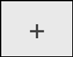button.

##### Subtraction
For subtraction, use the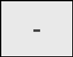symbol.
Note: This button can also be used to input the minus sign before a negative value.

##### Multiplication
For multiplication, use the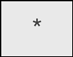symbol.
A multiplication symbol can be omitted when it occurs immediately before the opening parenthesis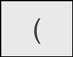or after the closing parenthesis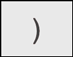.

3*(25.4 - 5) can be entered as 3(25.4 - 5)
(7 - 3.4)*(8 + 5.3) can be entered as (7 - 3.4)(8 + 5.3)
2*(4 - 8.03)*(6*7 - 10)*5 can be entered as 2(4 - 8.03)(6*7 - 10)5

5*x can also be entered as 5x
3*(x - 5) can be entered as 3(x - 5)
x*(5 - x) can be entered as x(5 - x)
(x + 3)*(x - 5) can be entered as (x + 3)(x - 5)
2*x(4 - 8*x)*(6*x - 10)*5 can be entered as 2x(4 - 8x)(6x - 10)5

##### Division
For division, use the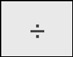button.

Note: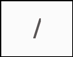symbol can be used for fractions input only. Do not use it for division.

##### Exponents
Exponents are typed using the power symbol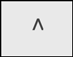Note: exponents can be positive or negative integers and expressions with the integer result. Exponents cannot be decimals.

Exponents of numbers:
32 would be typed as 3^2
(-5)8 as (-5)^8
4(-3) as 4^(-3)
7(5-3*2) as 7^(5-3*2).

Exponents of expressions:
(5+3-2.3*4)2 would be typed as (5+3-2.3*4)^2
(1.2*3-4)(-4) as (1.2*3-4)^(-4)

##### Parentheses
The calculator supports parentheses (), including nested parentheses.

Monday, December 4, 2023

Contact